Analysis Result Tables Created Edited

# Plate Force & Stress

## Function

• Check the internal forces and stresses of plate elements from the analysis results in a spreadsheet format table.
• Table Tool in midas Civil offers a variety of powerful built-in functions. Refer to Usage of Table Tool for detail directions.

## Call

From main menu, select [Results] tab > [Results Tables] group > [Plate] >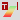[Force&Stress]

## Input

When you execute the Plate > Force & Stress Table function, the Records Activation Dialog is displayed. Here, you can select the target nodes, elements, load conditions, construction stages, and more, and then click the OK button. If you click Cancel, the table will be generated for all the targets, which may take a considerable amount of time depending on the case.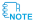Refer to Results Table of "Usage of Table Tool" for the usage of Records Activation Dialog.

Refer to Usage of Table Tool and check the following data:

1. Plate Force (Local)

1. Plate Force (Local)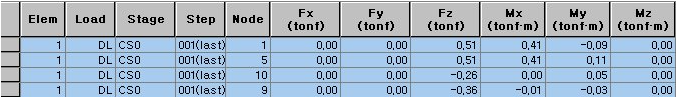Elem : Element number

Stage : Construction stage

Step : Sub-stage

Node : Node number

Fx : Element's internal force in the element's local x-direction

Fy : Element's internal force in the element's local y-direction

Fz : Element's internal force in the element's local z-direction

Mx : Bending moment about the element's local x-axis

My : Bending moment about the element's local y-axis

Mz : Bending moment about the element's local z-axis

2. Plate Force (Global)

2. Plate Force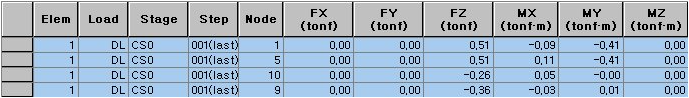Elem : Element number

Stage : Construction stage

Step : Sub-stage

Node : Node number

FX : Element's internal force in GCS X-direction

FY : Element's internal force in GCS Y-direction

FZ : Element's internal force in GCS Z-direction

MX : Bending moment about GCS X-axis

MY : Bending moment about GCS Y-axis

MZ : Bending moment about GCS Z-axisWhen performing moving load analysis, only the member forces per unit length are required. Therefore, when using the Plate Force (Local, Global) Table to output nodal forces, the results will be displayed as '0' since they are not relevant in this case.

3. Plate Force (Unit Length)

3. Plate Force (Unit Length)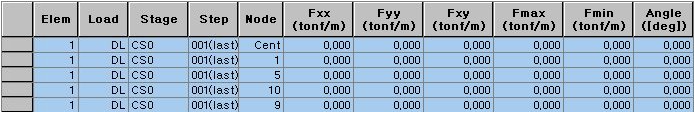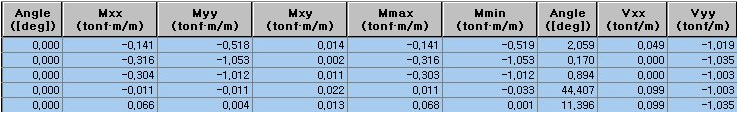Elem : Element number

Stage : Construction stage

Step : Sub-stage

Node : Node number

Fxx : Axial force per unit width in the element's local x-direction

Fyy : Axial force per unit width in the element's local y-direction

Fxy : Shear force per unit width in the element's local x-y plane (In-plane shear)

Fmax : Maximum principal axial force per unit width

Fmin : Minimum principal axial force per unit width

Angle : Angle formed by the element's local x-axis and the axis of the maximum principal axial force

Mxx : Bending moment per unit width in the x-axis direction of the element coordinate system.

Myy : Bending moment per unit width in the y-axis direction of the element coordinate system.

Mxy : Torsional moment per unit width about the element's local x-y axes

Mmax : Maximum principal bending moment per unit width

Mmin : Minimum principal bending moment per unit width

Angle : Angle formed by the element's local x-axis and the axis of the maximum principal bending moment

Vxx : Shear force per unit width in the thickness (z) direction on the element's local y-z plane

Vyy : Shear force per unit width in the thickness (z) direction on the element's local x-z plane

4. Plate Stress (Local)

4. Plate Stress (Local)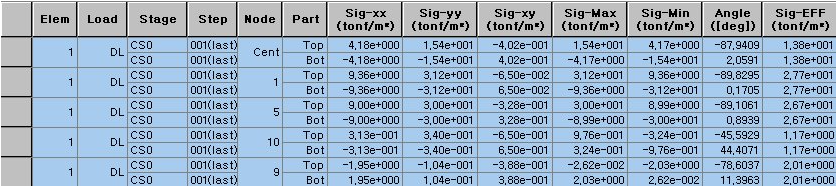Elem : Element number

Node : Node number

Stage : Construction stage

Step : Sub-stage

Part : Top or bottom fiber of a plate element in the element's local z-direction

Sig-xx : Axial stress in the element's local x-direction

Sig-yy : Axial stress in the element's local y-direction

Sig-xy : Shear stress in the element's local x-y plane (In-plane shear)

Sig-Max : Maximum principal stress

Sig-Min : Minimum principal stress

Angle : Angle formed by the element's local x-axis and the axis of the maximum principal stress vector. Angle is not generated for Envelop Type load combination since concurrent stresses cannot be calculated for the Envelop Type load combination.

Sig-EFF : Effective stress (von-Mises Stress)For the method of calculating principal stresses, effective stresses and maximum shear stresses for each load combination type, refer to the explanations at the bottom of the Combinations page.

5. Plate Stress (Global)

5. Plate Stress (Global)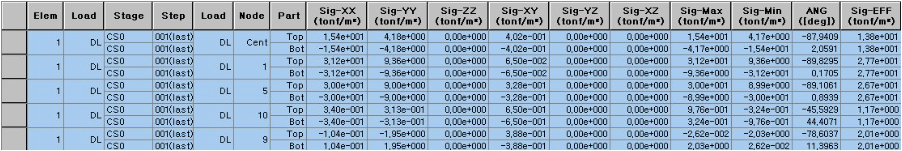Elem : Element number

Stage : Construction stage

Step : Sub-stage

Node : Node number

Part : Top or bottom fiber of a plate element in GCS Z-direction

Sig-XX : Axial stress in GCS X-direction

Sig-YY : Axial stress in GCS Y-direction

Sig-ZZ : Axial stress in GCS Z-direction

Sig-XY : Shear stress in GCS X-Y plane

Sig-YZ : Shear stress in GCS Y-Z plane

Sig-XZ : Shear stress in GCS X-Z plane

Sig-Max : Maximum principal stress

Sig-Min : Minimum principal stress

ANG : Angle formed by GCS X-axis and the axis of the maximum principal stress vector. Angle is not generated for Envelop Type load combination since concurrent stresses cannot be calculated for the Envelop Type load combination.

Sig-EFF : Effective stress (von-Mises Stress)The Stage and Step columns in the Analysis Result Table are produced for a construction stage analysis or Hydration Heat Analysis. The Step column is produced also for a geometric nonlinear analysis.
The calculation method for principal stress, von Mises stress, and maximum shear stress based on the load combination type is described in the "Combinations" section.

0